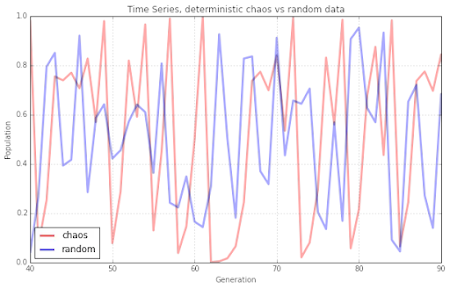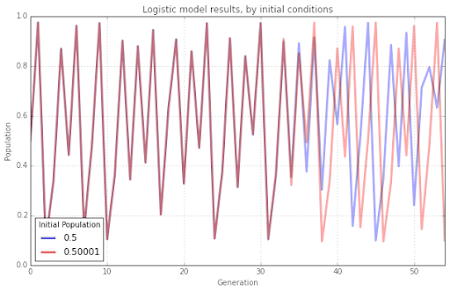### A chaotic balance

Our mathematical history begins in a discipline that, apparently, has very little to do with mathematics: biology. In 1975 on the journal Nature Robert May, an australian ecologist, publishes a review article with a rather indicative title: Simple mathematical models with very complicated dynamics(1). The heart of the paper is the following equation: $x_{t+1} = a x_t (1 - x_t)$ The equation, or logistic map, this is its name, describes the rate of change of a population in function of the parameter $t$ (the time), that varies in a discrete rather than continuous way, while $a$ is a constant that identifies the growth rate of a population. Insteed $x_t$ is the ratio between the existing population and the maximum possible population at time $t$.
The model thus described is deterministic, i.e. the population at instant 0 determines the population at subsequent instants. The equation predicts the existence of a stationary state, i.e. a situation in which the population at time $t + 1$ is equal to the population at time $t$. This state is stable, that is, it is maintained for a sufficiently long time, but only for $a$ lower than or equal to 3. However when the growth rate exceeds this value, the size of the population begins to oscillate between 0 and 1, apparently in a random way. But if we observe carefully, we notice small more or less periodic recurrences, which show how the behavior of the equation is actually chaotic.Differences between chaotic and random behavior - via Geoff Boeing)
And because the equation describes the growth of a population quite well, the conclusion is quite obvious: equilibrium, in nature, does not exist, or at least there is no stable condition in nature. It is also possible to clearly see the so-called butterfly effect. This is typically told with the story of the flapping butterfly in Brazil that generates a tornado in New York. In mathematical terms, the flapping of the butterfly's wings is a variation, even a small one, of the initial conditions, for example of the population size at time 0. For example, a variation of just 1/10000 generates a variation that begins to become evident from the 30th generation.The butterfly effect in a single plot - via Geoff Boeing)
In fact, May had started to deal with this topic in 1974 when Biological Populations with Nonoverlapping Generations: Stable Points, Stable Cycles, and Chaos(2) was published on Science. In this paper he presented some simple models of the growth of biological populations. In addition to some differential equations, May also presented a variation on the logistic map.
There are many examples of the presence of chaos in the most disparate systems, for example in the three-body problem, in general all of them can be studied within the theory of chaos.
1. May, R. M. (1976). Simple mathematical models with very complicated dynamics. Nature, 261(5560), 459-467. doi:10.1038/261459a0 ↩︎
2. May, R. M. (1974). Biological populations with nonoverlapping generations: stable points, stable cycles, and chaos. Science, 186(4164), 645-647. doi:10.1126/science.186.4164.645 ↩︎